## Equilibrium

The Equilibrium Concept Builder includes 36 questions organized into 12 Question Groups and spread across three difficulty levels. Each question involves the same task - add an east-west vector and a north-south vector to an object so that it is in a state of equilibrium. Questions in the same difficulty level include the same number of given vectors. Questions in the same Question Group are very similar, having the same number of given vectors to be balanced and having those vectors in the same quadrants.

The three difficulty levels are differentiated as follows:

• Apprentice Difficulty Level Questions 1-4: A single vector is given. The vector makes an angle to the traditional coordinate axes. Students must add an E/W vector and a N/S vector to balance the force.
• Master DifficultyLevel Questions 5-8: Two vectors are given. The vectors make an angle to the traditional coordinate axes. Students must add an E/W vector and a N/S vector to balance the forces.
• Wizard Difficulty Level Questions 9-12: Three vectors are given. The vectors make an angle to the traditional coordinate axes. Students must add an E/W vector and a N/S vector to balance the forces.

The Physics Classroom grants teachers and other users the right to print these questions for private use. Users are also granted the right to copy the text and modify it for their own use. However, this document nor its graphics should not be uploaded to other servers for distribution to and/or display by others. The Physics Classroom website should remain the only website or server from which the document  and its graphics is distributed or displayed. We also provide a PDF that teachers can use under the same conditions. We have included a link to the PDF near the bottom of this page.

#### Equilibrium

Apprentice Difficulty Level
Question Group 1
Question 1
Consider the angled force below. Add one E-W force and one N-S force so that the object is at equilibrium.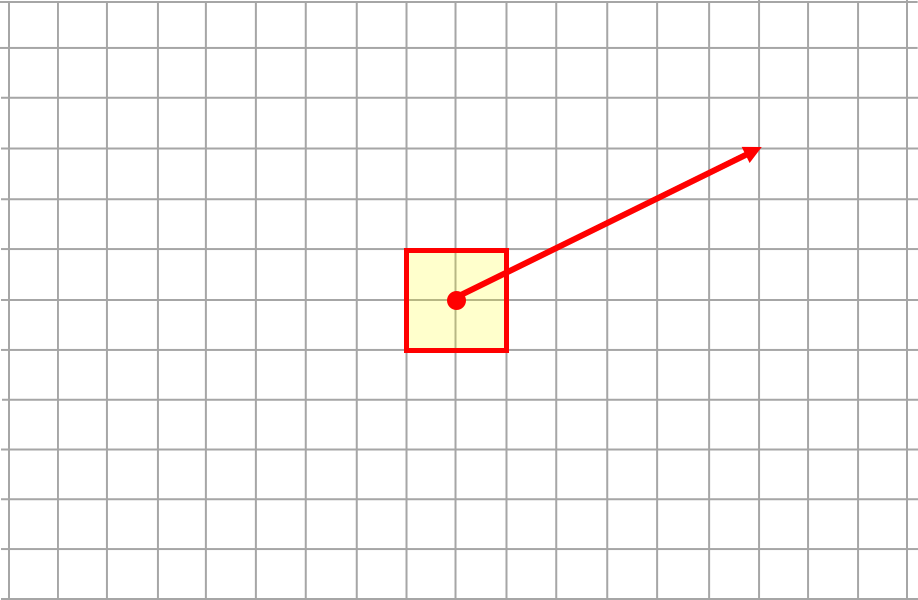Question 2
Consider the angled force below. Add one E-W force and one N-S force so that the object is at equilibrium.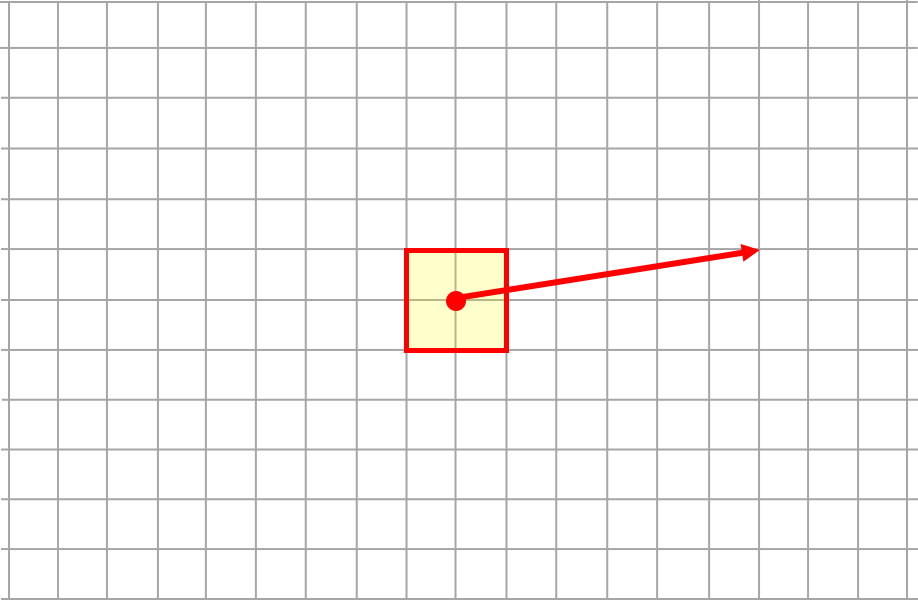Question 3
Consider the angled force below. Add one E-W force and one N-S force so that the object is at equilibrium.Question Group 2
Question 4
Consider the angled force below. Add one E-W force and one N-S force so that the object is at equilibrium.Question 5
Consider the angled force below. Add one E-W force and one N-S force so that the object is at equilibrium.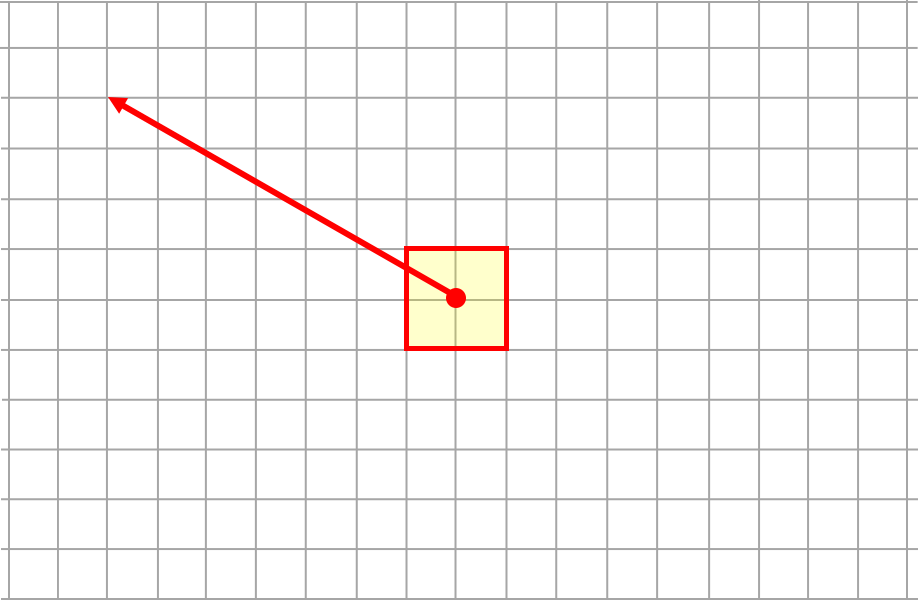Question 6
Consider the angled force below. Add one E-W force and one N-S force so that the object is at equilibrium.Question Group 3
Question 7
Consider the angled force below. Add one E-W force and one N-S force so that the object is at equilibrium.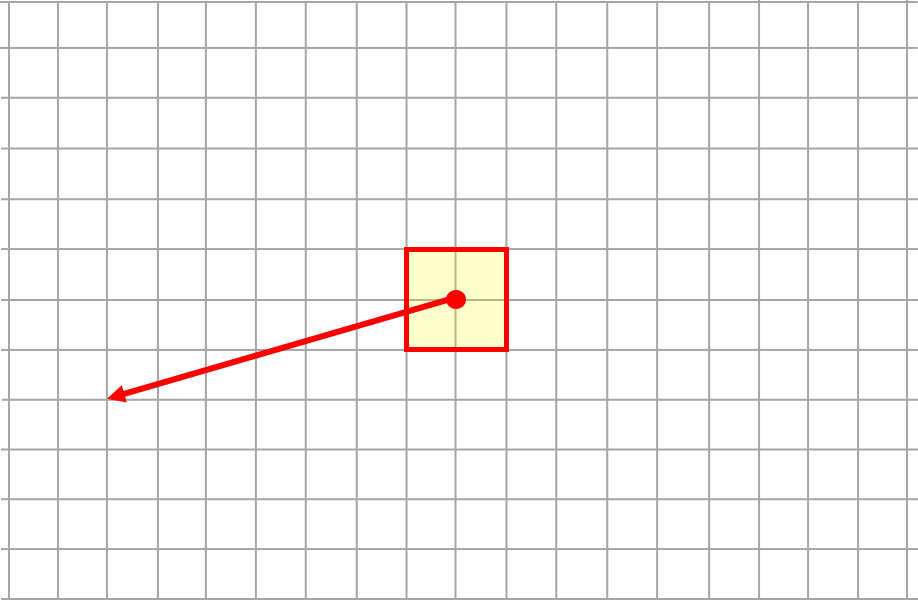Question 8
Consider the angled force below. Add one E-W force and one N-S force so that the object is at equilibrium.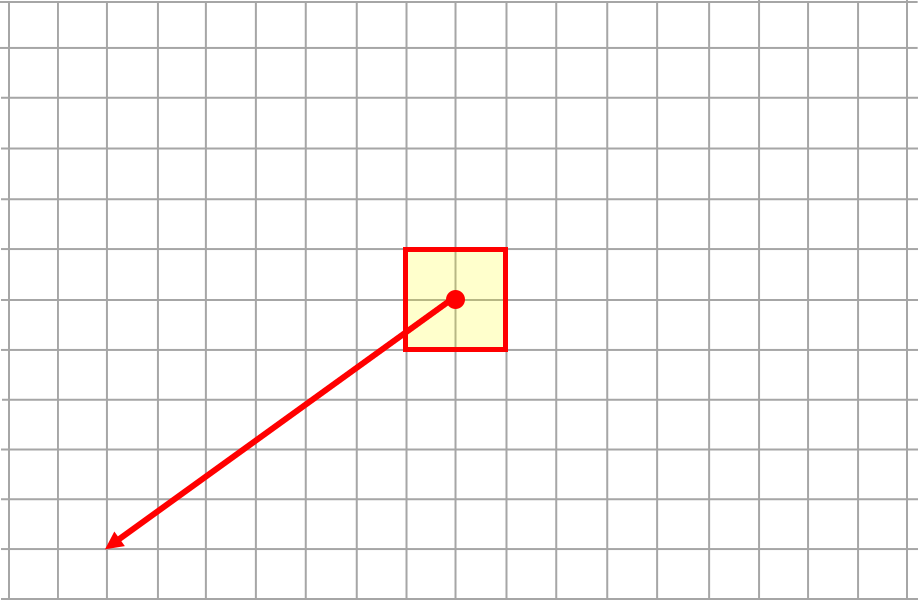Question 9
Consider the angled force below. Add one E-W force and one N-S force so that the object is at equilibrium.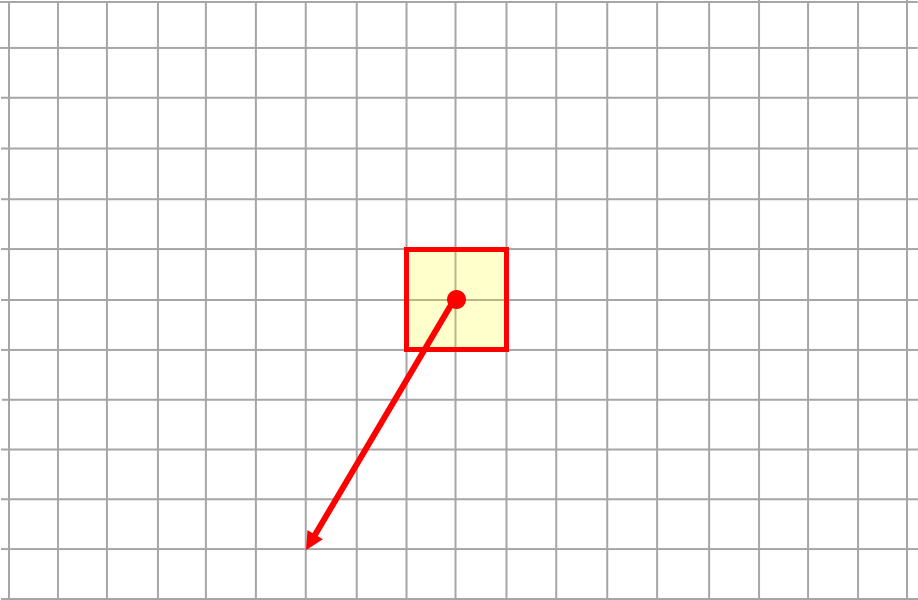Question Group 4
Question 10
Consider the angled force below. Add one E-W force and one N-S force so that the object is at equilibrium.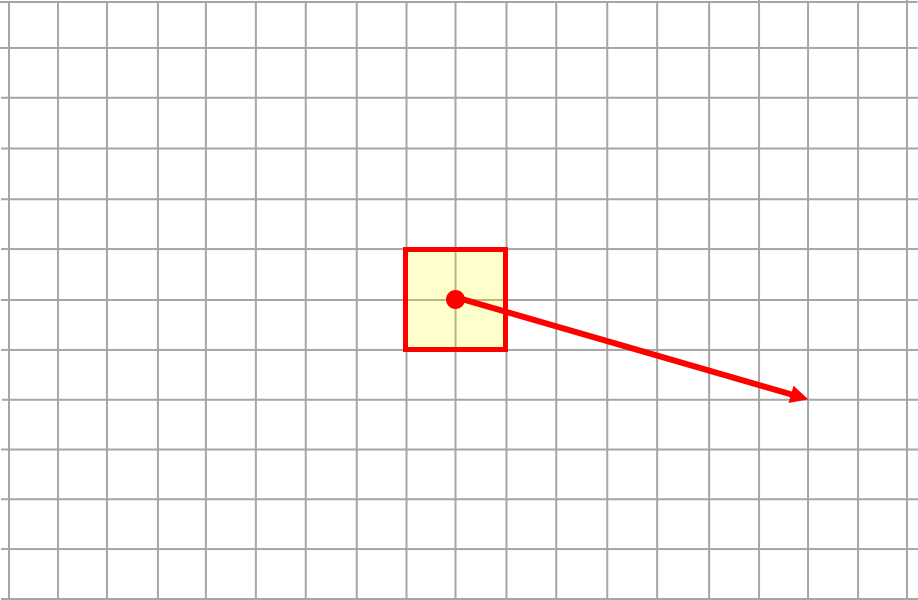Question 11
Consider the angled force below. Add one E-W force and one N-S force so that the object is at equilibrium.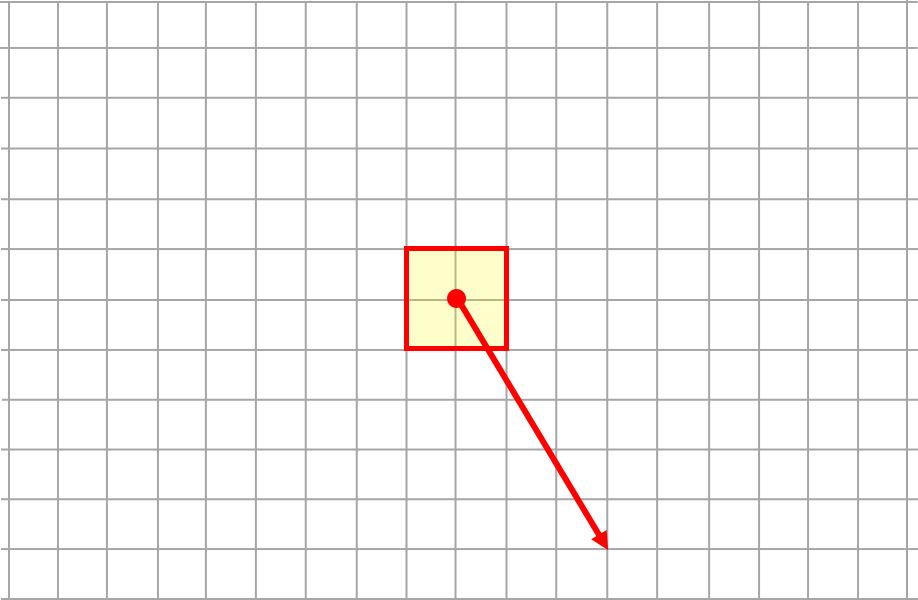Question 12
Consider the angled force below. Add one E-W force and one N-S force so that the object is at equilibrium.Master Difficulty Level
Question Group 5
Question 13
Consider the two angled forces below. Add one E-W force and one N-S force so that the object is at equilibrium.Question 14
Consider the two angled forces below. Add one E-W force and one N-S force so that the object is at equilibrium.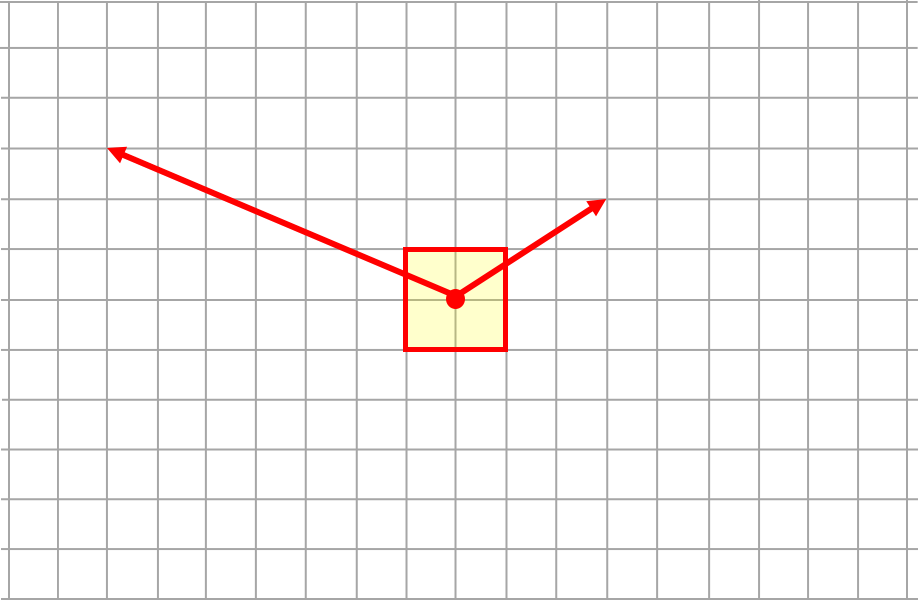Question 15
Consider the two angled forces below. Add one E-W force and one N-S force so that the object is at equilibrium.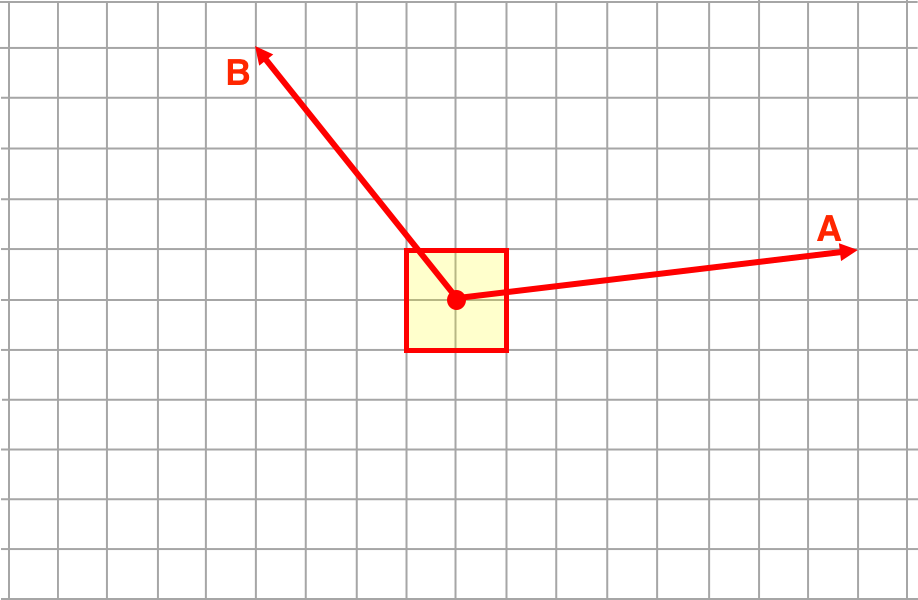Question Group 6
Question 16
Consider the two angled forces below. Add one E-W force and one N-S force so that the object is at equilibrium.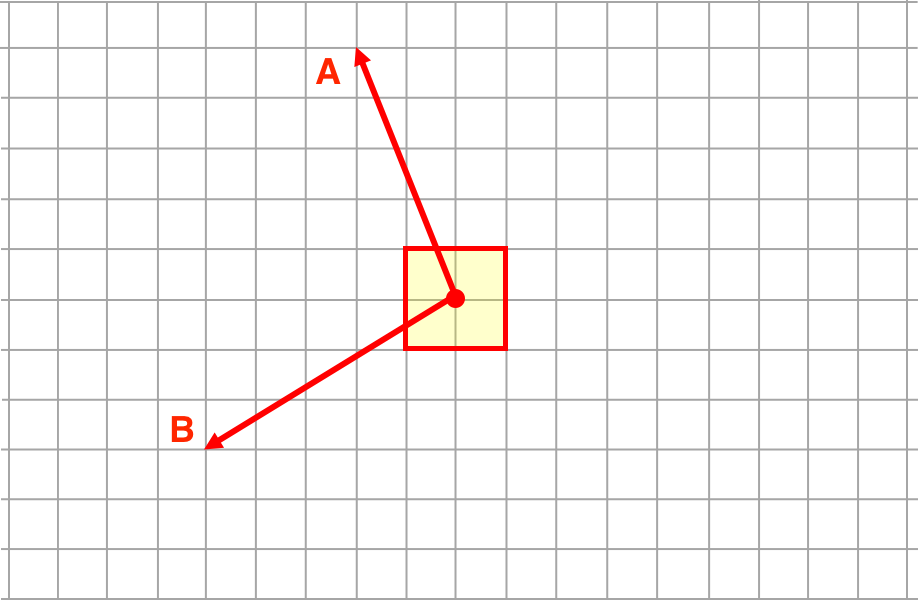Question 17
Consider the two angled forces below. Add one E-W force and one N-S force so that the object is at equilibrium.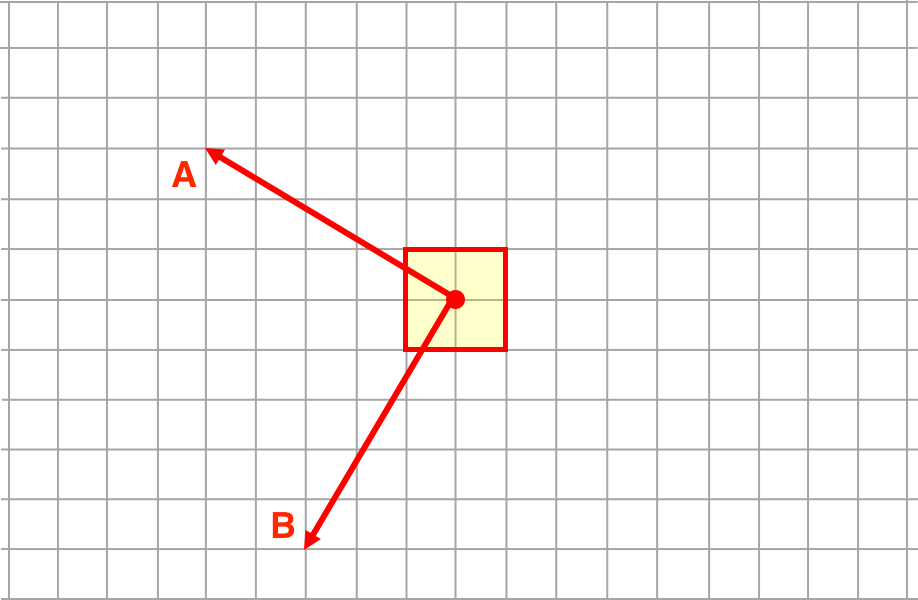Question 18
Consider the two angled forces below. Add one E-W force and one N-S force so that the object is at equilibrium.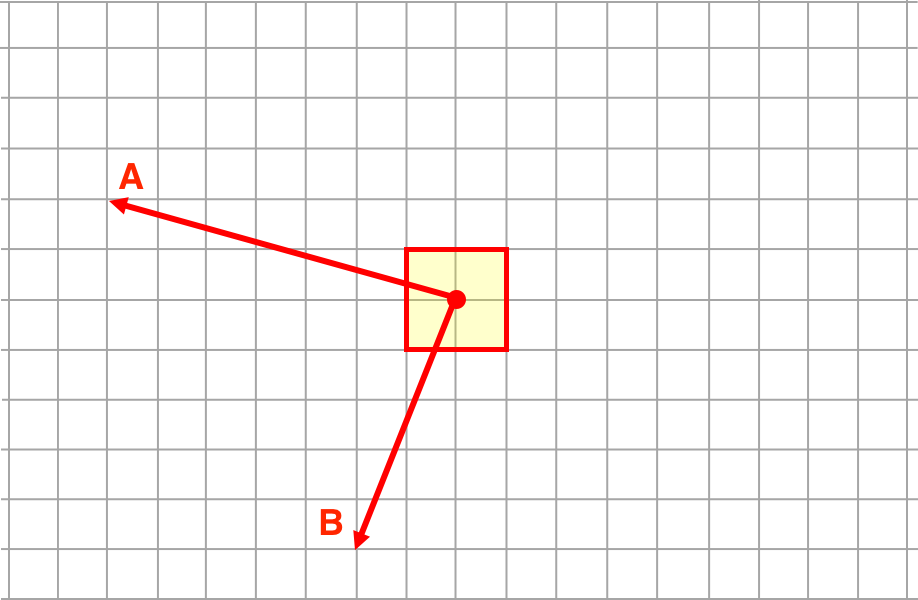Question Group 7
Question 19
Consider the two angled forces below. Add one E-W force and one N-S force so that the object is at equilibrium.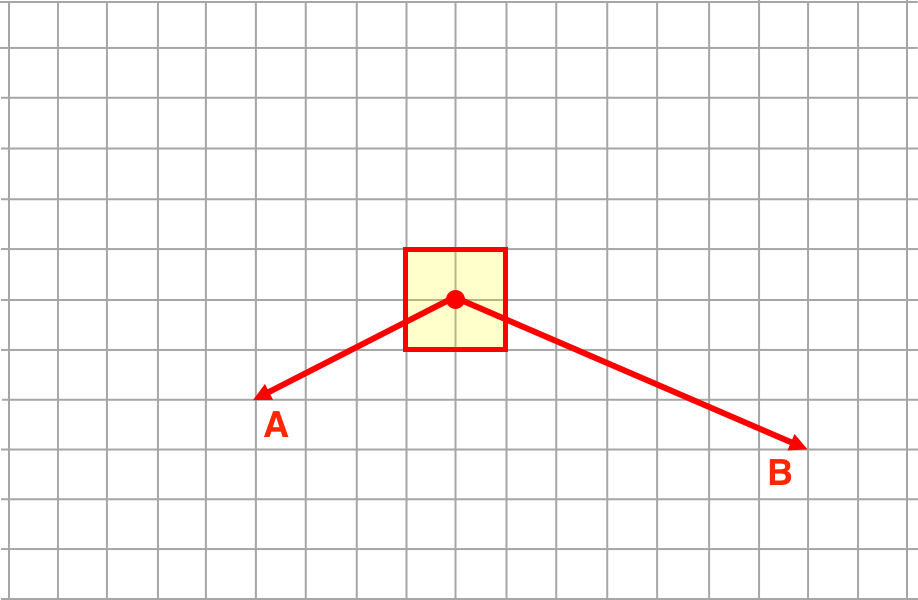Question 20
Consider the two angled forces below. Add one E-W force and one N-S force so that the object is at equilibrium.Question 21
Consider the two angled forces below. Add one E-W force and one N-S force so that the object is at equilibrium.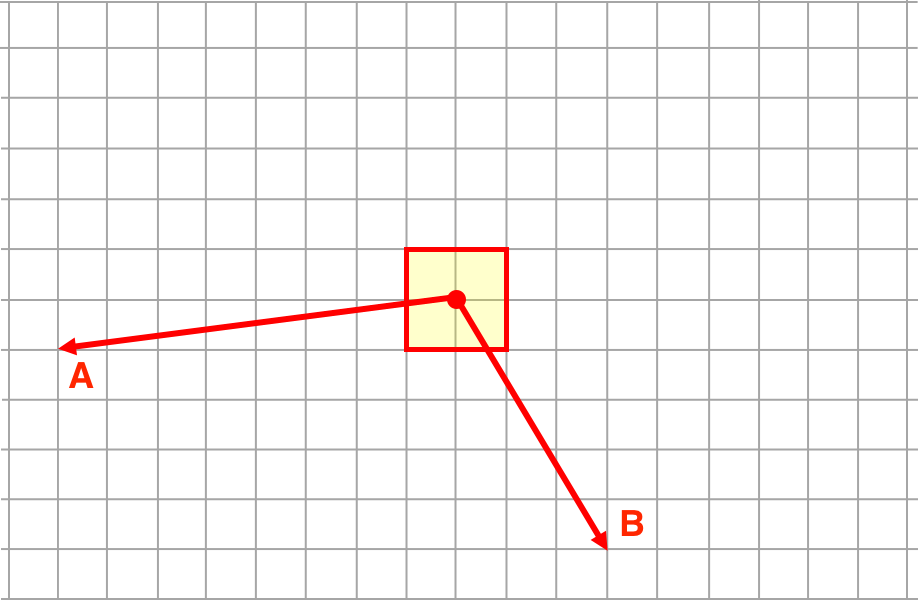Question Group 8
Question 22
Consider the two angled forces below. Add one E-W force and one N-S force so that the object is at equilibrium.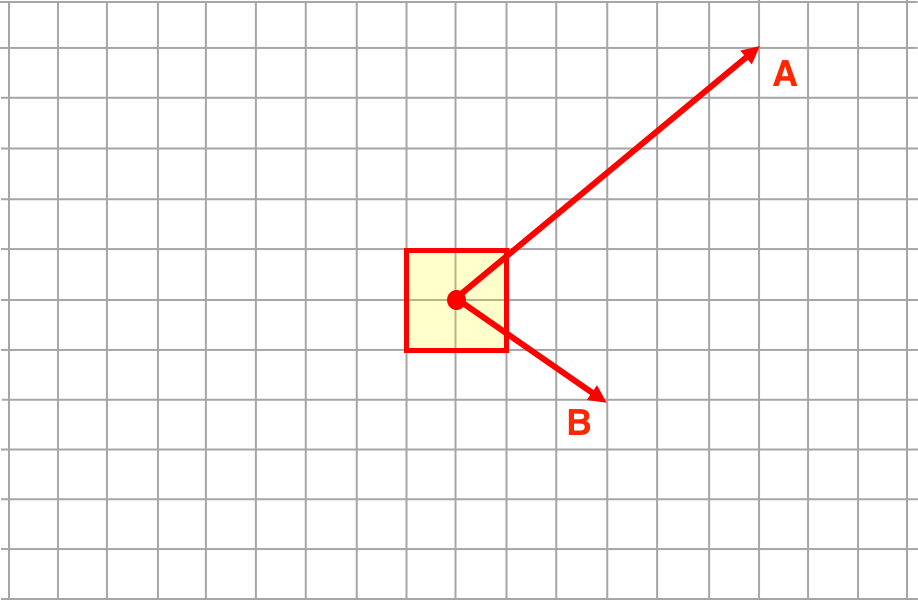Question 23
Consider the two angled forces below. Add one E-W force and one N-S force so that the object is at equilibrium.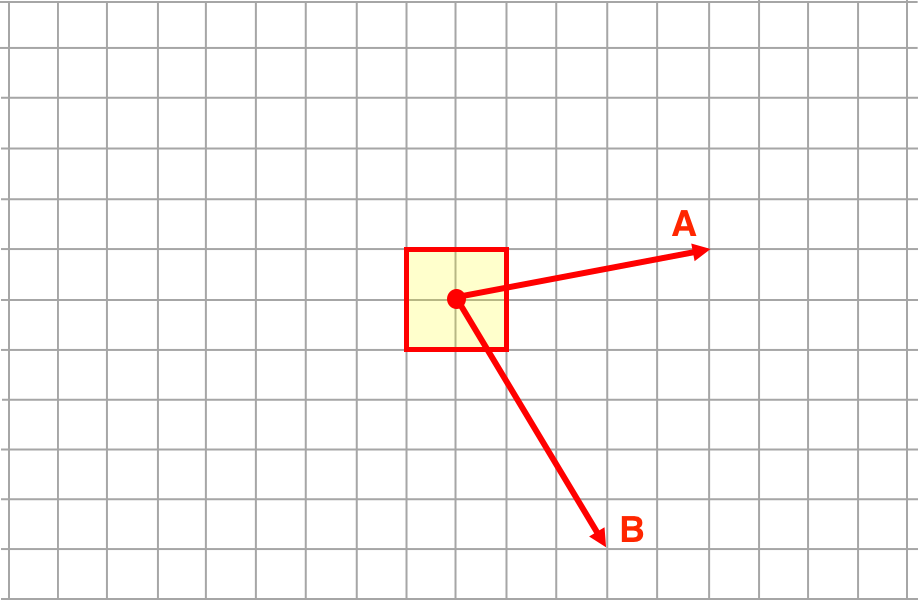Question 24
Consider the two angled forces below. Add one E-W force and one N-S force so that the object is at equilibrium.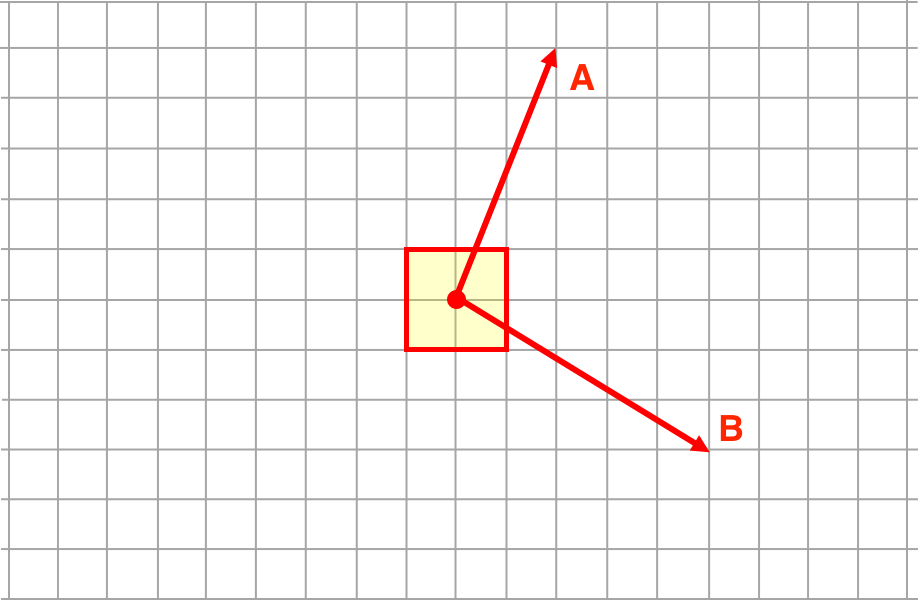Wizard Difficulty Level
Question Group 9
Question 25
Consider the three angled forces below. Add one E-W force and one N-S force so that the object is at equilibrium.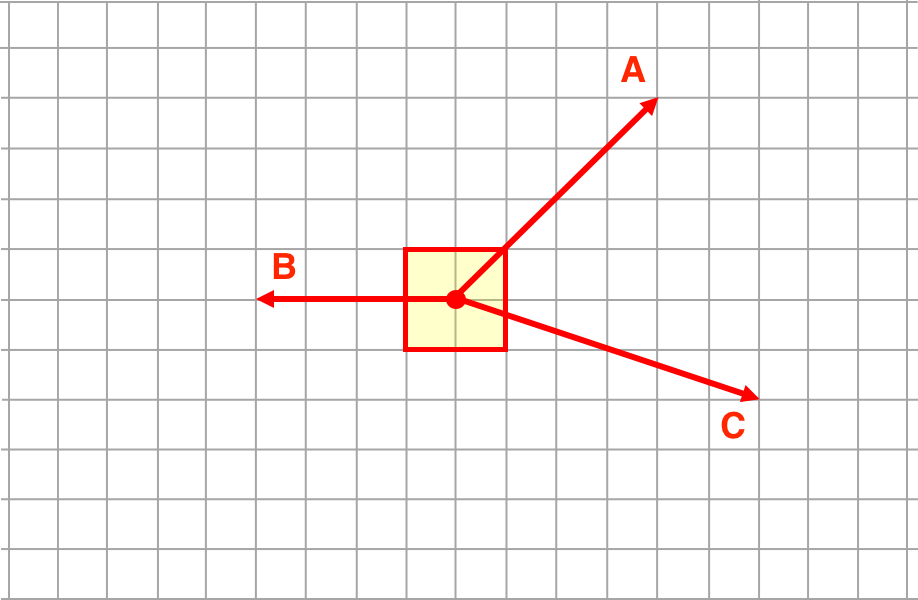Question 26
Consider the three angled forces below. Add one E-W force and one N-S force so that the object is at equilibrium.Question 27
Consider the three angled forces below. Add one E-W force and one N-S force so that the object is at equilibrium.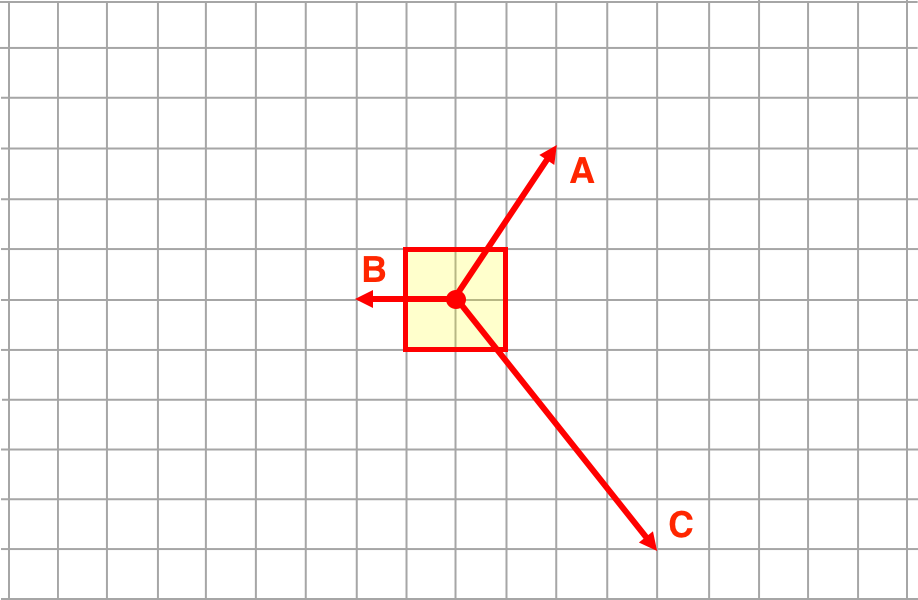Question Group 10
Question 28
Consider the three angled forces below. Add one E-W force and one N-S force so that the object is at equilibrium.Question 29
Consider the three angled forces below. Add one E-W force and one N-S force so that the object is at equilibrium.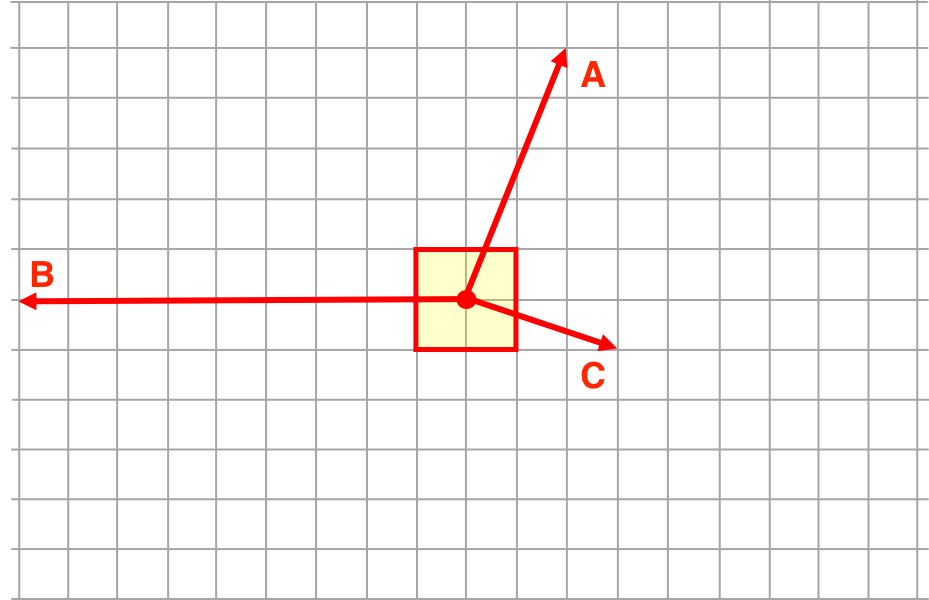Question 30
Consider the three angled forces below. Add one E-W force and one N-S force so that the object is at equilibrium.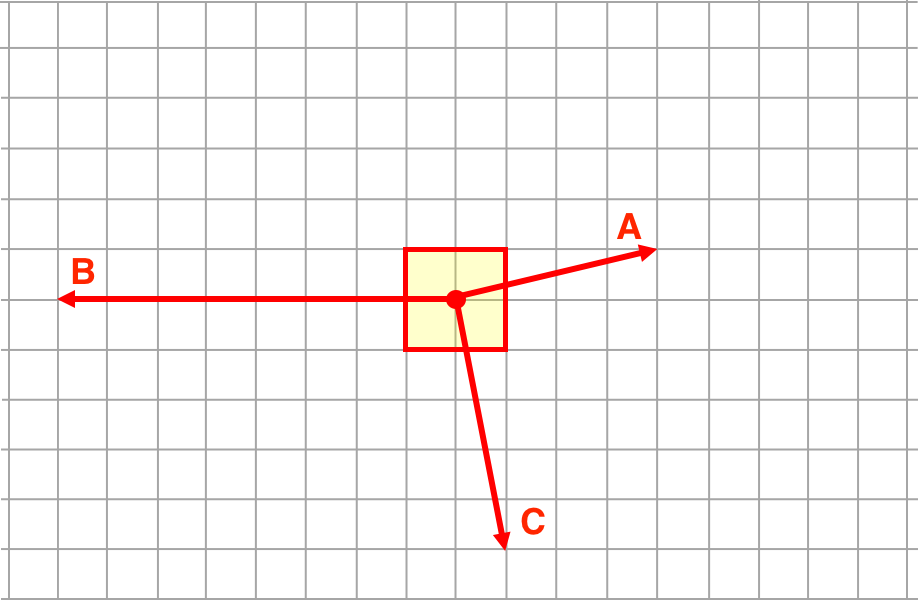Question Group 11
Question 31
Consider the three angled forces below. Add one E-W force and one N-S force so that the object is at equilibrium.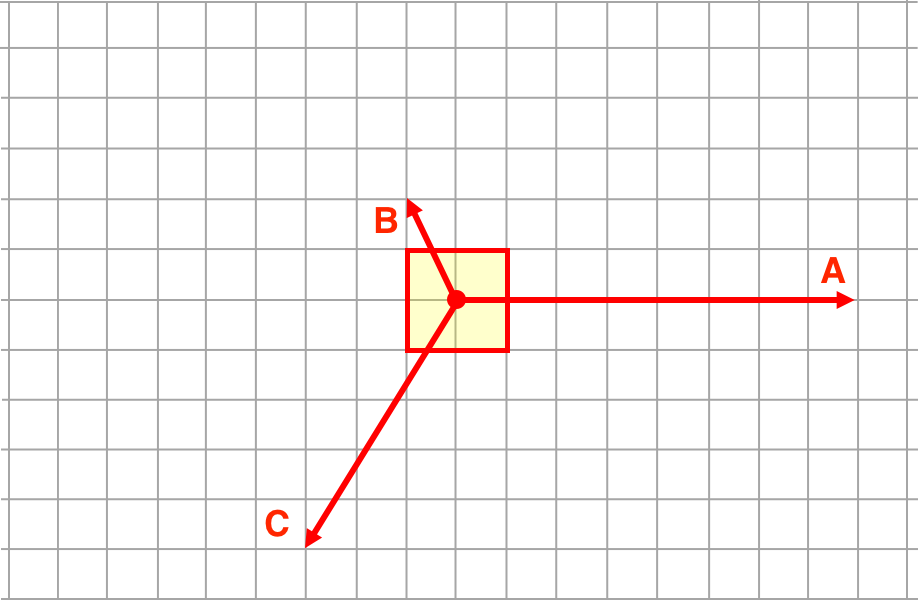Question 32
Consider the three angled forces below. Add one E-W force and one N-S force so that the object is at equilibrium.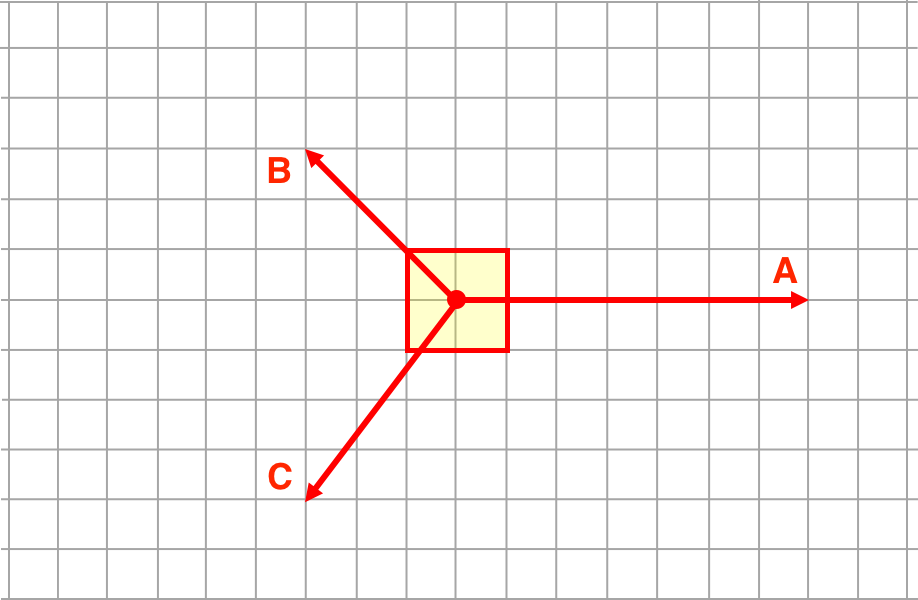Question 33
Consider the three angled forces below. Add one E-W force and one N-S force so that the object is at equilibrium.Question Group 12
Question 34
Consider the three angled forces below. Add one E-W force and one N-S force so that the object is at equilibrium.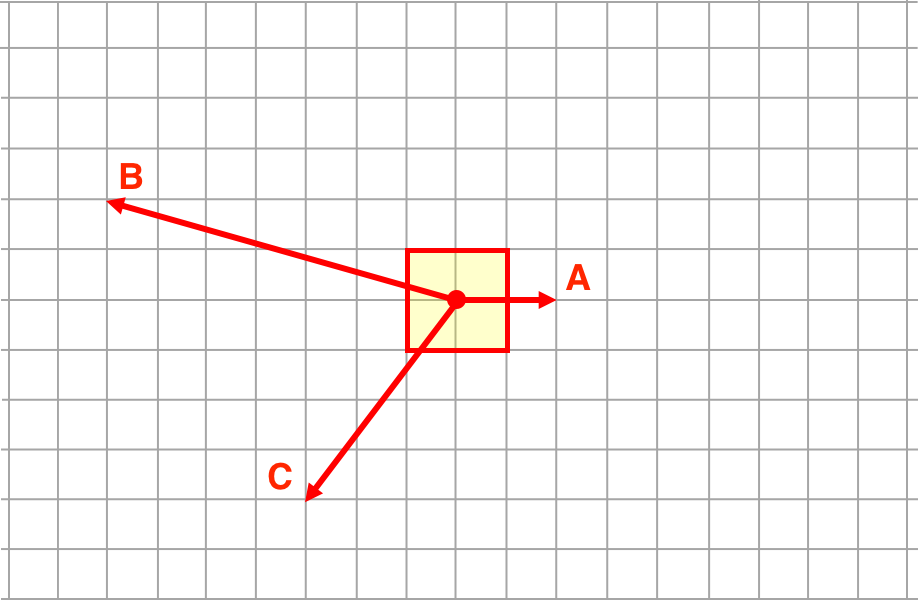Question 35
Consider the three angled forces below. Add one E-W force and one N-S force so that the object is at equilibrium.Question 36
Consider the three angled forces below. Add one E-W force and one N-S force so that the object is at equilibrium.View:  Questions in PDF Format

Visit: Concept Builder || Teacher Notes || Directions